期刊菜单

A Fast Algorithm for Short Term Scheduling of Cascaded Hydropower Reservoir Systems
DOI: 10.12677/JWRR.2018.73026, PDF, HTML, XML, 下载: 1,140  浏览: 3,744

Abstract: With the development of hydropower systems, more and more complex demands are encountered by hydropower scheduling. To obtain near optimal schedules of hydropower systems effectively without solving complex mathematic programming, a fast algorithm is proposed. Without an objective function, the basic scheduling concepts of saving water, servicing for power system balance, coordination of up and down stream reservoirs, together with most of the constrains of optimization models, are used to direct the scheduling of cascaded hydropower systems. The method is composed of a series of operations for whole time horizon requirements such as final reservoir level, total energy or total turbine water of a plant, total energy of several cascaded reservoirs, and reducing spill water. To achieve one target, a successive power scheduling method is proposed in which temporal order of power rise/fall based on load characters and a local scheduling algorithm that can satisfy period coupled constraints are combined. Case study of hydropower system in Hongshui River operated by China Southern Power Grid (CSG) shows that the proposed method is effective in obtaining power schedules and complex power distribution rules can be achieved.

1. 引言

2. 建模及求解方法

2.1. 水电调度控制目标

1) 水库期末蓄水量/水位：

${S}_{m}^{T+1}=S{T}_{m}={S}_{m}^{1}+\underset{t=1}{\overset{T}{\sum }}\left({I}_{m}^{t}-{R}_{m}^{t}\right)$ (1)

2) 水库发电水量：

${W}_{m}=\underset{t=1}{\overset{T}{\sum }}\left(3600{q}_{m}^{t}{\Delta }_{t}\right)$ (2)

3) 水电站发电量：

${E}_{m}=\underset{t=1}{\overset{T}{\sum }}\left({p}_{m}^{t}{\Delta }_{t}\right)$ (3)

4) 水电站群的总电量(可选)：

$Esy{s}_{n}=\underset{m\in {U}_{n}}{\sum }\underset{t=1}{\overset{T}{\sum }}\left({p}_{m}^{t}{\Delta }_{t}\right)$ (4)

2.2. 其他水电调度约束

1) 水量平衡约束：

${S}_{m}^{t+1}={S}_{m}^{t}+{I}_{m}^{t}-{R}_{m}^{t}$ (5)

2) 发电流量约束：

${q}_{m}^{t}\le {\stackrel{¯}{q}}_{m}^{t}$ (6)

3) 水电站出力约束：

${\underset{_}{p}}_{m}^{t}\le {p}_{m}^{t}\le {\stackrel{¯}{p}}_{m}^{t}$ (7)

4) 库容限制：

${\underset{_}{S}}_{m}^{t}\le {S}_{m}^{t}\le {\stackrel{¯}{S}}_{m}^{t}$ (8)

5) 水电站出力爬坡限制：

$|{p}_{m}^{t}-{p}_{m}^{t-1}|\le {\stackrel{¯}{\Delta p}}_{m}$ (9)

6) 出库流量限制：

${\underset{_}{R}}_{m}^{t}\le {R}_{m}^{t}\le {\stackrel{¯}{R}}_{m}^{t}$ (10)

7) 水电站振动区约束：

$\left[{p}_{m}^{t}-{\stackrel{¯}{ps}}_{m,k}\left({H}_{m}^{t}\right)\right]\left[{p}_{m}^{t}-{\underset{_}{ps}}_{m,k}\left({H}_{m}^{t}\right)\right]\ge 0$ (11)

8) 水电站出力波动限制：

$\left({p}_{m}^{t-\Delta }-{p}_{m}^{t-\Delta -1}\right)\left({p}_{m}^{t}-{p}_{m}^{t-1}\right)\ge 0,\text{}\Delta =1,2,\cdots ,t{v}_{m}-1$ (12)

9) 最小出力升降时段数限制：

m号水电站出力从上升开始至下降开始，或者由下降开始至上升开始的时间间隔不少于 $t{p}_{m}$ 个时段。此约束是通过限制出力升降的间隔时间，提高调度计划可执行性。定义状态变量 ${X}_{m}^{t}$ 描述出力升降时段数，

${X}_{m}^{t}=\left\{\begin{array}{l}\mathrm{min}\left({X}_{m}^{t-1}+1,t{p}_{m}\right)\text{}\text{\hspace{0.17em}}\text{}\left({p}_{m}^{t}-{p}_{m}^{t-1}\right)\ge 0\text{and}{X}_{m}^{t-1}>0\\ 1\text{}\text{\hspace{0.17em}}\text{}\text{ }\text{ }\text{ }\left({p}_{m}^{t}-{p}_{m}^{t-1}\right)>0\text{and}{X}_{m}^{t-1}<0\\ \mathrm{max}\left({X}_{m}^{t-1}-1,-t{p}_{m}\right)\text{}\text{\hspace{0.17em}}\text{}\left({p}_{m}^{t}-{p}_{m}^{t-1}\right)\le 0\text{and}{X}_{m}^{t-1}<0\\ -1\text{}\text{\hspace{0.17em}}\text{}\left({p}_{m}^{t}-{p}_{m}^{t-1}\right)<0\text{and}{X}_{m}^{t-1}>0\text{}\end{array}$

$\left(|{X}_{m}^{t-1}|-t{p}_{m}\right){X}_{m}^{t}{X}_{m}^{t-1}\le 0$ (13)

2.3. 时段排序规则

1) 水电站库容足够时，水电站出力需要优先承担系统高峰负荷，然后分配到负荷平坦阶段，最后分配到低谷阶段。根据系统负荷特点预设峰、平、谷时段  ；

2) 上游水电站出库流量应考虑下游水电站机组过流能力和库容限制，避免弃水；

3) 对于梯级水电站群，各水电站间可以相互协调负荷尖峰(高峰负荷阶段的最高负荷)时段的出力，如单一水电站在不同尖峰负荷时段的出力比例可以设置；

4) 所有水电站的运行应该避免在振动区和高水耗区。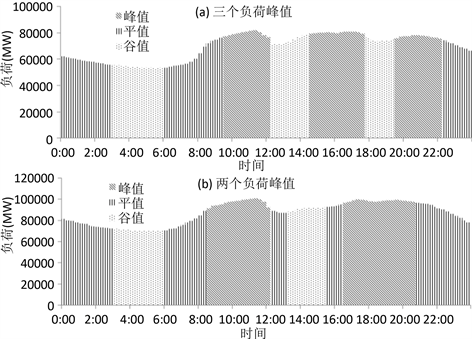Figure 1. Distribution of peak, flat, and valley stages

UPn，UFn，UVn，UMn分别是第n个峰、平、谷、尖峰阶段包括的时段集合。NP，NF，NV，NM分别是峰、平、谷、尖峰负荷的阶段数量。当NP = NM时，每个高峰负荷阶段只有一个尖峰。 ${w}_{m}^{t}$ 为描述水电站m在时段t增加出力优先级的变量， $-{w}_{m}^{t}$ 描述减少出力的优先级。

${w}_{m}^{t}={a}_{0}\phi \left(t\right)+{a}_{1}{C}^{t}-{a}_{2}{\sum }_{m0=1}^{M}{p}_{m0}^{t}+\gamma \left(m,t\right)$ (14)

$\phi \left(t\right)=\left\{\begin{array}{l}vp\text{}t\in U{P}_{n},\text{}n=1,2,\cdots ,NP\\ vf\text{}t\in U{F}_{n},\text{}n=1,2,\cdots ,NF\\ vv\text{}t\in U{V}_{n},\text{}n=1,2,\cdots ,NV\end{array}$ (15)

$\gamma \left(m,t\right)=\left\{\begin{array}{l}{a}_{3}\left(\frac{{p}_{m}^{t}}{\underset{t0\in UM}{\sum }{p}_{m}^{t0}}-{r}_{m}^{n}\right)\text{}t=U{M}_{n},n=1,2,\cdots ,NM\\ 0\text{others}\end{array}$ (16)

2.4. 可行性修正算法

$\left\{\begin{array}{l}\text{Ob}\text{.max}y1\\ \text{S}\text{.T}\text{.}\left\{\begin{array}{l}\left({p}_{m}^{t}-{p}_{m}^{t+1}\right)\left({p}_{m}^{t}-{p}_{m}^{y1}\right)\le \text{0}\\ y1=t-t{v}_{m}+1,t-t{v}_{m}+2,\cdots ,t-1\end{array}\end{array}$ (17)

$\left\{\begin{array}{l}\text{Ob}\text{.min}y2\\ \text{S}\text{.T}\text{.}\left\{\begin{array}{l}\left({p}_{m}^{t+1}-{p}_{m}^{t}\right)\left({p}_{m}^{y2}-{p}_{m}^{t}\right)\le \text{0}\\ y1=t+2,t+3,\cdots ,t+t{v}_{m}\end{array}\end{array}$ (18)

2.5. 满足单一调度需求的方法

2.5.1. 当前水库调度计划修改方法

1) 获取潜在的影响范围。设定 $ts=1$ 。如果需在时段 $\left[t1,t2\right]$ 内增加蓄水量，就需要减少该时段之前的出库水量。找到t1前的最后一个水库水位达到上限的时段，并将ts重新设定为此值。因为在水位达到上限的时段之前若减小部分时段出库，将使得水位达到上限时段附近的出力变化导致时间关联约束破坏，或者出现弃水，需要对此进一步修正而使得计算非常复杂。同理，如果在时段 $\left[t1,t2\right]$ 内需要减少蓄水量，将ts设定为t1前的最后一个库水位达到下限的时段；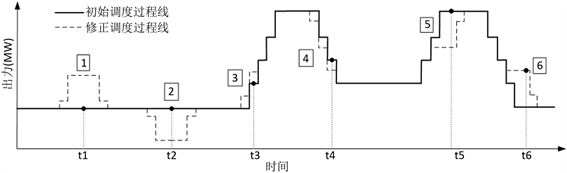Figure 2. Examples for power modification at adjacent periodsTable 2. Influencing combinations for requirements

2) 将 $\left[ts,t1-1\right]$ 中的时段储存在集合U中，在最小出力(或最小发电流量)约束下减小出库实现t1时段蓄水量增加；在最大出力(或最大发电流量)约束下增加出库实现蓄水量减小；

3) 将集合U中的时段根据时段排序规则排序，按顺序储存为 $\left\{{u}_{1},{u}_{2},\cdots ,{u}_{n}\right\}$ ，并设定 $k=1$

4) 以设定步长增减时段 ${u}_{k}$ 的出力值，采用可行性修改算法获得新的可行出力过程。保持水库m的直接下游水库出库流量过程不变，水库m及其下游水库按照新的调度计划进行模拟。新的调度计划可能会造成水库弃水量增加，最小出力或最小发电流量的约束破坏，水位超过限制等。若发生如上情况，那么对原调度计划的修改是无效的，重新设定 $k=k+\text{1}\le n$ 并重新执行步骤4)；

5) 如果步骤4)中调度计划修改成功，但仍没有达到蓄水量增减目标，则返回步骤1)，否则停止。

2.5.2. 相邻水库调度计划修改方法

1) 设定 $ts=1$ 。如果水库 $m+1$ 蓄水量在时段 $\left[t1,t2\right]$ 需要增加，找到t1前的最后一个水库 $m+1$ 水位达到上限时的初始时段，并将ts设定为此值。同理，若要在时段内需要减少蓄水量，则将ts设定为t1前的最后一个水库 $m+1$ 水位达到下限的时段；

2) 考虑到上下游水流滞时，设定水库m可调整时段为 $\left[t{s}^{\prime },t2-{\underset{_}{l}}_{m}\right]$$t{s}^{\prime }=ts-{\stackrel{¯}{l}}_{m}$${\stackrel{¯}{l}}_{m}$ 为水库m到水库 $m+1$ 之间水流最大滞时时段数。如果水库 $m+1$$\left[t1,t2\right]$ 内需要增加蓄水量，即水库m在 $\left[t{s}^{\prime },t2-{\underset{_}{l}}_{m}\right]$ 内需要加大出库，在 $\left[t{s}^{\prime },t2-{\underset{_}{l}}_{m}\right]$ 内找到水库m最后一个水位达到下限时的时段，将 $t{s}^{\prime }$ 设定为此值。同理，若水库 $m+1$$\left[t1,t2\right]$ 内需要减少蓄水量，则将 $t{s}^{\prime }$ 设定为 $\left[t{s}^{\prime },t2-{\underset{_}{l}}_{m}\right]$ 内最后一个水库m的库水位达到上限的时段。将 $\left[t{s}^{\prime },t2-{\underset{_}{l}}_{m}\right]$ 的时段储存在集合U中；

3) 将集合U中的时段进行排序，按顺序储存为 $\left\{{u}_{1},{u}_{2},\cdots ,{u}_{n}\right\}$ ，并设定 $k=1$

4) 以设定步长修改水库m在时段 ${u}_{k}$ 的出力值，采用可行修正算法获得新的调度过程。保持水库 $m+1$ 的出库流量不变，对水库m及其下游水库 $m+1$ 按照新的调度计划进行模拟。如果新的调度计划导致弃水量增加，约束越界等情况，那么计划修改失败，重新设定 $k=k+\text{1}\le n$ 并重新执行步骤4)；

5) 如果步骤4) 中调度计划修改成功，但没有达到蓄水量的增减目标，则返回步骤1)，否则停止。

2.6. 梯级水库群发电计划制定

2.6.1. “自上而下”与“自下而上”的计算模式

1) 将编号 $m=1$ 的水库设置为当前水库；

2) 不考虑对下游水库 $m+1$ 的影响，对水库m进行出力分配以满足其控制目标；

3) 使用“自上而下”模式来分配水库m的出力，以达到减少其弃水并满足最小出力与最小流量约束的目的；如果上游存在 $m-1$ 水库，必要的情况下采用“自上而下”模式改变水库 $m-1$ 的出力过程减小水库m的弃水并满足最小出力和最小流量约束的目标；

4) 若水库 $m-1$ 由于步骤3)偏离控制目标，则利用“自上而下”模式修改水库 $m-1$ 的出力。如果水库m的约束限制了水库 $m-1$ 的出力修改，则反复进行时段排序并对水库m在排序靠前的时段进行出力增减和可行性修正，以逐步引导水库 $m-1$ 通过“自下而上”模式满足水库m新的出力过程的用水，同时使得水库 $m-1$ 接近控制目标；

5) 如果在步骤4)之后水库m的控制目标出现偏差，则用“自上而下”模式进行再次修正m的调度计划；

6) 设定 $m=m+1$ ，如果 $m>M$ 执行步骤7)，否则执行步骤2)；

7) 检查水库是否仍有控制目标无法满足。如果不能同时满足上、下游水库的控制目标，则需根据预先设定的目标优先级，采用“自上而下”模式或“自下而上”模式对其进行修正。

2.6.2. 整体协调分配出力模式

1) 计算 ${e}_{m}^{t}=\frac{\partial {E}_{storage}}{\partial {p}_{m}^{t}}$ 的值，其中 ${E}_{storage}$ 是系统蓄能，短期调度中 ${e}_{m}^{t}$ 值变化幅度一般很小，近似所有 ${e}_{m}^{t}$ 为一个值 ${e}_{m}$

2) 将所有水库出力设为最小值，从上游到下游进行减少弃水的操作；

3) 计算电量差 $\Delta E\text{=}{\sum }_{m=1}^{M}\underset{t=1}{\overset{T}{\sum }}\left({p}_{m}^{t}{\Delta }_{t}\right)-Esy{s}_{1}$ ，若其绝对值小于给定精度转到步骤8)；

4) 按时段排序规则进行排序，设定 $l=1$

5) 如果 $\Delta E>0$ ，将水库根据 ${e}_{m}$ 的值降序排序；如果 $\Delta E<0$ ，将水库根据 ${e}_{m}$ 的值升序排序。设定 $k=1$

6) 在时段 ${u}_{l}$ 附近修改水库k的出力，如果电量差的绝对值减小，转至步骤3)，否则设定 $k=k+1$ ，如果 $k\le M$ ，重新执行步骤6)，否则执行步骤7)；

7) 设定 $l=l+1$ ，如果 $l\le T$ ，执行步骤5)。如果系统总电量较步骤4)开始时没有变化，转到步骤8)，否则转至步骤4)；

8) 停止。

3. 实例研究

3.1. 红水河梯级水电站

3.1.1. 实例1，给定各水库控制目标Table 3. Basic parameters of the reservoirsFigure 3. Schematic layout of studied hydropower system(a) 均匀调峰模式 (b) 比例调峰模式 (c) 出力与负荷一致模式

Figure 4. Comparison for different peak shaving mode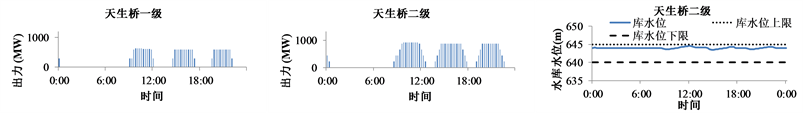(a) 天生桥一级总电量5000 MWh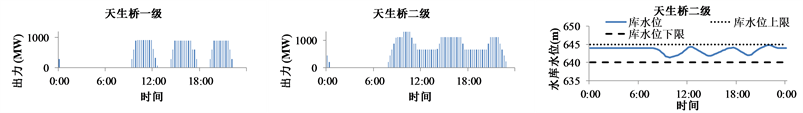(b) 天生桥一级总电量7500 MWh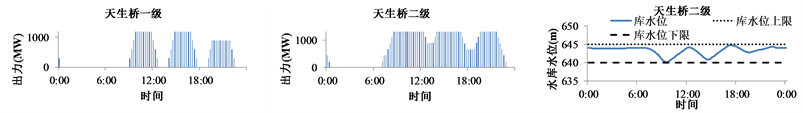(c) 天生桥一级总电量10,000 MWh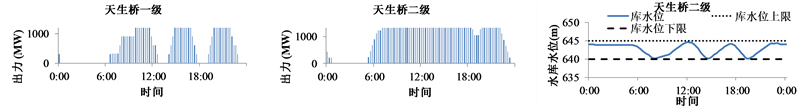(d) 天生桥一级总电量12,500 MWh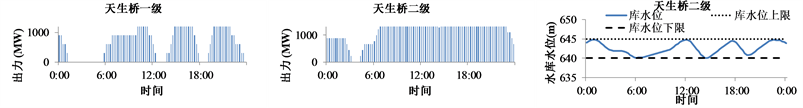(e) 天生桥一级总电量15,000 MWh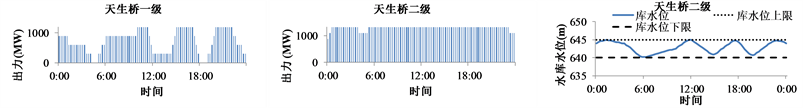(f) 天生桥一级总电量17,500 MWh

Figure 5. Schedules given energy for TSQ-1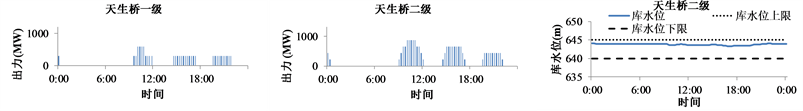(a) 天生桥二级总电量5000 MWh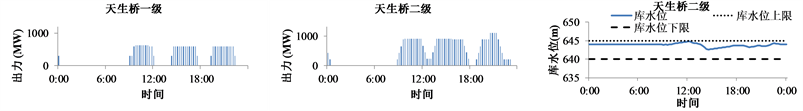(b) 天生桥二级总电量10,000 MWh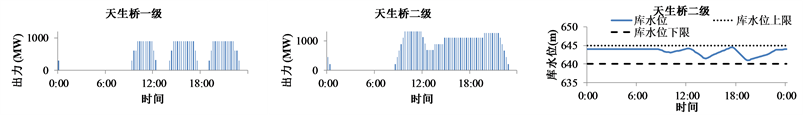(c) 天生桥二级总电量15,000 MWh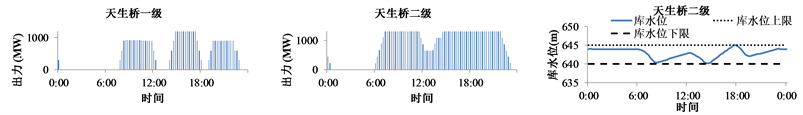(d) 天生桥二级总电量20,000 MWh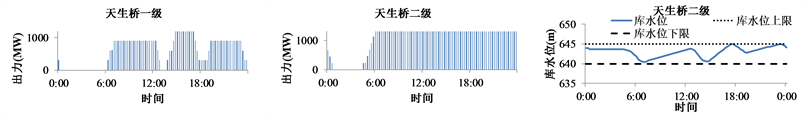(e) 天生桥二级总电量25,000 MWh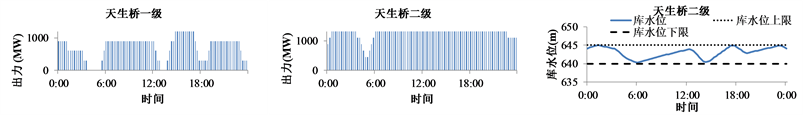(f) 天生桥二级总电量30,000 MWh

Figure 6. Schedules given energy for TSQ-2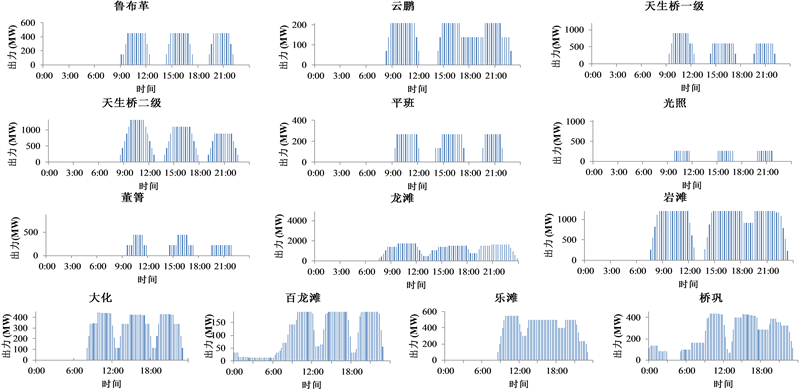(a) 各水库出力过程(b) 各日调节水库水位过程

Figure 7. Schedules of plants subjected to reservoir control targets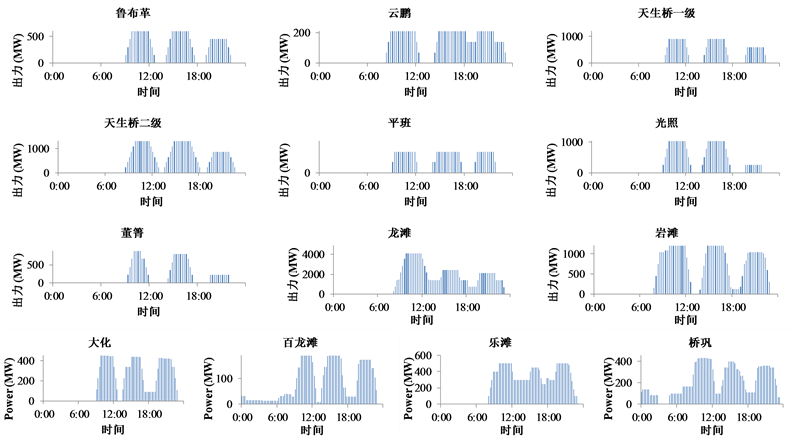(a) 各水库出力过程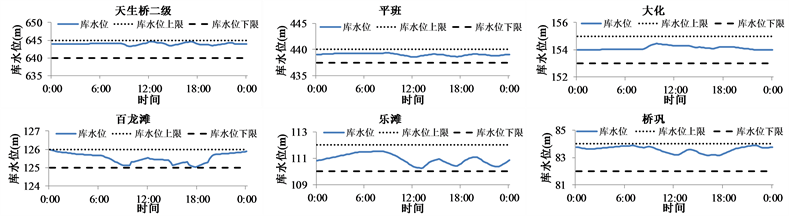(b) 各日调节水库水位过程

Figure 8. Schedules of plants subjected to target of cascaded system energy

3.1.2. 实例2，给定系统发电量控制目标

4. 结论